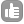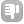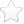3Why the chemical shift range of 1H nmr is ~20 ppm wheras for 13C nmr it is ~230 ppm? asked Jan 12 '11 at 15:58Evgeny Fadeev 577●1 Sameer, I've edited your title a little bit to clarify. - Evgeny Fadeev (Jan 13 '11 at 12:24)3Chemical shift is the slight change in resonance frequency of the nucleus based on the magnetic field that the nucleus "sees" through its electron cloud... thus the smaller the magnitude of change of this electron cloud, the smaller the range of chemical shift. Hydrogen has one electron and when it bonds its electron density can't change but by so much. For example, if Hydrogen is bonded to a very electronegative atom, like F, F essentially pulls away that electron, but Hydrogen's electron density has only changed by a little less than its original electron. If a F is bonded to a Carbon, however, its electron density will change a lot more. answered Jan 14 '11 at 11:28very good! - nmr (Jan 19 '11 at 04:35)1Shielding differs. 1 ppm = 0.0001% Some nuclei can have 10000 ppm = 1% of difference in chemical environment... answered Jan 19 '11 at 03:161The chemical shift in solution (a single number) derives from the averaging of the chemical shift tensor (nine elements) which reflects the change in resonance frequency with the angle of orientation of the tensor to the external magnetic field. The tensor encapsulates the effect of the shielding provided to the nucleus by the electron distribution around the nucleus. I am not expert in this area but you can easily imagine that the polarisability of the electrons around a given nucleus and the asymmetry of the elctron distrubution around the nucleus is going to be a sensitive function of the available 'chemistry' for that atom and the extent to which the bonding electrons are sensed at the nucleus. The asymmetry aspect is important - leading to the concept known as chemical shift anisotropy (CSA) - and is most evident in NMR spectra acquired for molecules in the solid state (the diagonal tensor components correlate with the shape of the powder pattern for example -- again not my speciality). answered Feb 02 '11 at 06:281the ppm scale is defined as the ratio between a variation of resonance frequency and a reference resonance frequency. The ppm range of 13C is bigger than ppm range of 1H because little perturbation of electronic environment of 13C is bigger compared to the major contribution of Larmor frequency. The gyromagnetic ratio is 4 times lower for 13C. So if the perturbation is identical for 1H and 13C, the range have to be 4 times bigger. We see that is 10 times bigger, so an other contribution exist, likely a more susceptibility of electronic density to be disturbed... answered Feb 07 '11 at 14:00Yoan Monneau 1360Chemical shift is dependent on The nucleus type, and the electron density in the vicinity. answered Jan 13 '11 at 06:14j 131 Actually, the RANGE is dependent on nucleus type...... But the chemical shift itself is not dependent on nucleus type, since it is of course normalized to a reference nucleus. - j (Jan 14 '11 at 09:06)0If you consider that the Hydrogen atom has only one electron around the nucleus, then the chemical shifts for 13C can be of as much as 6 times larger range since Carbon has 6 electrons around its nucleus. If the Proton range is multiplied by 6 it would correspond to 6 times the range at Proton gyromagnetic ratio. Or 10 ppm at 100MHz (for proton) corresponds to 1 KHz frequency spread. For 6 times it would be 6KHz or 6000Hz. Carbon resonance frequency correspondingly (for the same magnetic field strength) would be only 25 MHz. Which means 1ppm=25 Hz. When the frequency spread 6 x 1000 = 6000 is divided by 25 Hz, 6000/25= 4 x 60 = 240 ppm range which is the 13C chemical shift range in ppm. This looks very simple; may be that this work out simple enough for 13C,nd not for other nuclei. On the similar basis we can try out a simple procedure for which we have to sit together and sort out matters. Aravamudhan answered Feb 14 '11 at 06:32toggle preview

Tags:

×26
×12
×8
×1

Asked: Jan 12 '11 at 15:58

Seen: 17,631 times

Last updated: Feb 15 '11 at 11:23